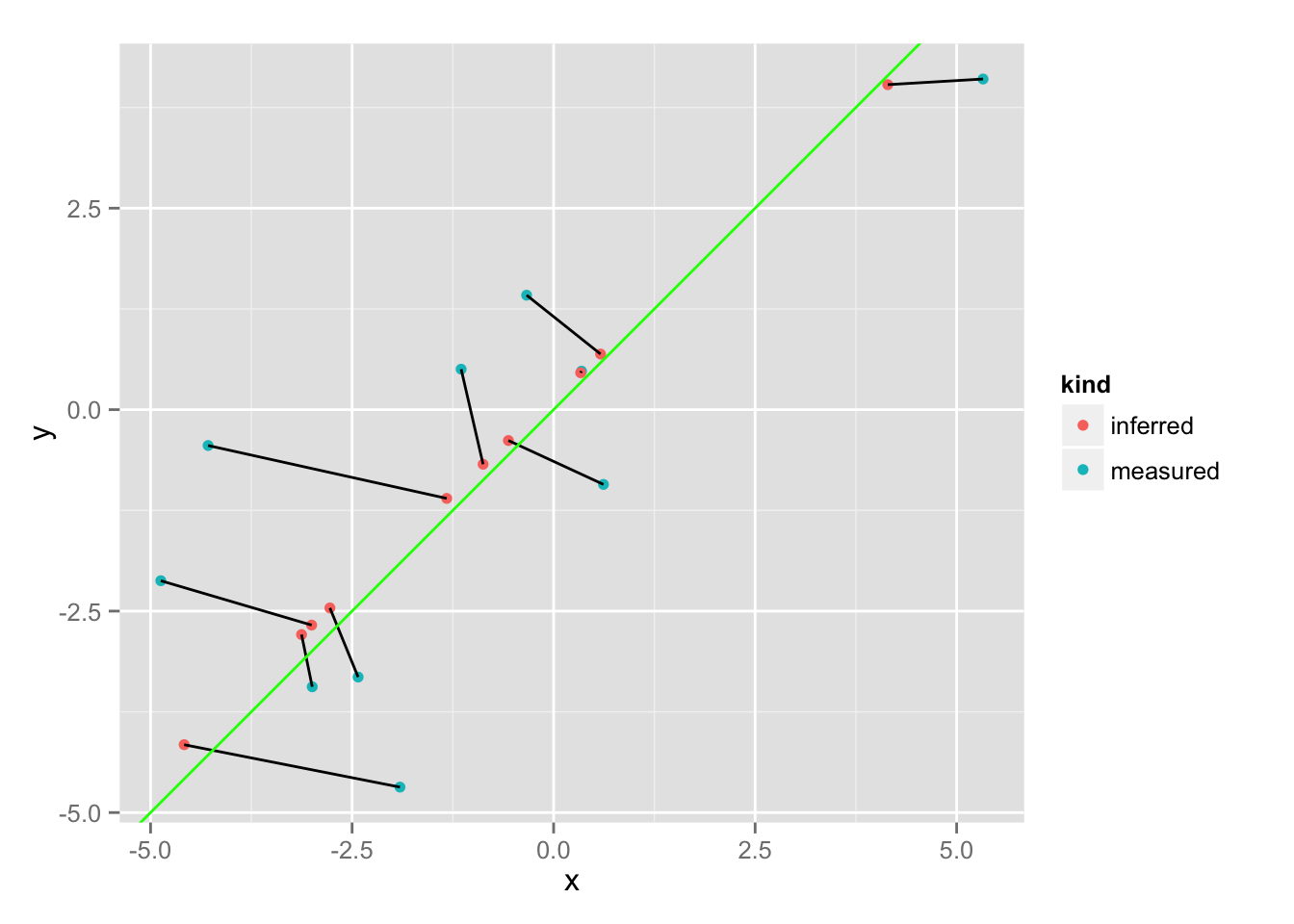## Tuesday, June 14, 2016

### My knitr LaTeX template: manuscript and supplement interleaved in one source file

Most of the time between starting manuscript and having it accepted after peer-review is spent writing, re-writing and re-arranging content. In Word, keeping track of figure numbers is a big pain, even more so when figures are moved between the main manuscript and the supplement. Moving my Word manuscript to knitr, I first had to decide between RMarkdown and LaTeX. Adding citations and figure references in RMarkdown seems still a bit experimental, and I decided to go with LaTeX.

For my template, I implemented two main features. First, thanks to a tip by Iddo Friedberg, supplemental figures are automatically numbered "S1", "S2", etc. Second, I added a bit of LaTeX magic to interleave parts of the main manuscript and the supplement: If I want to move a paragraph or figure to the supplement, I just wrap it with a "\supplement{ }" command. That is, in the source code, the main and supplemental text are right next to each other, and only in the generated PDF are they separated into two parts of the document.

Of course, fulfilling journal requirements once the manuscript is accepted might still take some time, but compared to the time saved in the previous stages this is quite acceptable.

Here is the template, and here an example PDF generated from the template. (For a complete manuscript, see here.)

## Tuesday, April 19, 2016

### Display element ids for debugging Shiny apps

My current Shiny project contains at least five tables and I constantly forget how they are called. So I whipped up a little bookmarklet that uses jQuery to show the id of each div and input. Some of those can be ignored as they are internal names set by Shiny, but most are the actual names you define in R.

## Friday, March 11, 2016

### New R package: a dictionary with arbitrary keys and values

Coming from Python, the absence of a real dictionary in R has annoyed me for quite some time. Now, I actually needed to use vectors as keys in R:
```library(dict)

d <- dict()

d[] <- 42
d[[c(2, 3)]] <- "Hello!"
d[["foo"]] <- "bar"
d[]
d[[c(2, 3)]]
d\$get("not here", "default")

d\$keys()
d\$values()
d\$items()

# [[ ]] gives an error for unknown keys
d[["?"]]
```

Under the hood, separate C++ dictionaries (unordered_map) are created for the different types of keys. Using R's flexible types that are reflected in Rcpp (as SEXP), such a dictionary can store both numbers and strings (and other objects) at the same time.

The package is available on GitHub: https://github.com/mkuhn/dict

## Tuesday, March 8, 2016

### Avoiding unnecessary memory allocations in R

As a rule, everything I discover in R has already been discussed by Hadley Wickham. In this case, he writes:
The reason why the C++ function is faster is subtle, and relates to memory management. The R version needs to create an intermediate vector the same length as y (x - ys), and allocating memory is an expensive operation. The C++ function avoids this overhead because it uses an intermediate scalar.
In my case, I want to count the number of items in a vector below a certain threshold. R will allocate a new vector for the result of the comparison, and then sum over that vector. It's possible to speed that up about ten-fold by directly counting in C++:

Often this won't be the bottleneck, but may be useful at some point.

## Monday, August 24, 2015

### Fitting with uncertainty using Bayesian inference

This is a mirror of my post on RPubs. The RMarkdown source can be found here.

In this toy example, we assume that we’ve independently measured values \(x\) and \(y\) and want to find a linear relationship between them, accounting for measurement uncertainty. Each \(x\) and \(y\) value is assigned a different uncertainty, and the challenge is to take this information into account. A standard linear model will treat all points equally.

When we treat each measurement as a multivariate normal distribution, we can find a point along the proposed fitted line that is maximizing the probability density function (i.e. that has a maximum likelihood). Thus, given a slope and intercept, we virtually move all measurements to their most likely point along the line, and use the likelihood at this point.

``library(rstan)``
``````## Loading required package: Rcpp
##
## Attaching package: 'inline'
##
## The following object is masked from 'package:Rcpp':
##
##     registerPlugin
##
## rstan (Version 2.7.0-1, packaged: 2015-07-17 18:12:01 UTC, GitRev: 05c3d0058b6a)
## For execution on a local, multicore CPU with excess RAM we recommend calling
## rstan_options(auto_write = TRUE)
## options(mc.cores = parallel::detectCores())``````
``````library(ggplot2)

set.seed(42)

N <- 10``````

We create 10 points:

``````x0 <- seq(-5, 5, 10.1/N)
y0 <- x0

sigma_x <- abs( rnorm( length(x0), 1) )
x <- x0 + unlist(lapply(sigma_x, function(sigma) rnorm(1, 0, sigma)))

sigma_y <- abs( rnorm( length(y0), 1) )
y <- y0 + unlist(lapply(sigma_y, function(sigma) rnorm(1, 0, sigma)))

data <- data.frame( x, sigma_x, y, sigma_y )

data_list <- list( x=x, sigma_x=sigma_x, y=y, sigma_y = sigma_y, N=N)

ggplot( data, aes(x, y) ) + geom_point() + geom_abline(color="red") + geom_smooth(method="lm", se=F) + geom_errorbar(aes(ymin=y-sigma_y, ymax=y+sigma_y)) + geom_errorbarh(aes(xmin=x-sigma_x, xmax=x+sigma_x))``````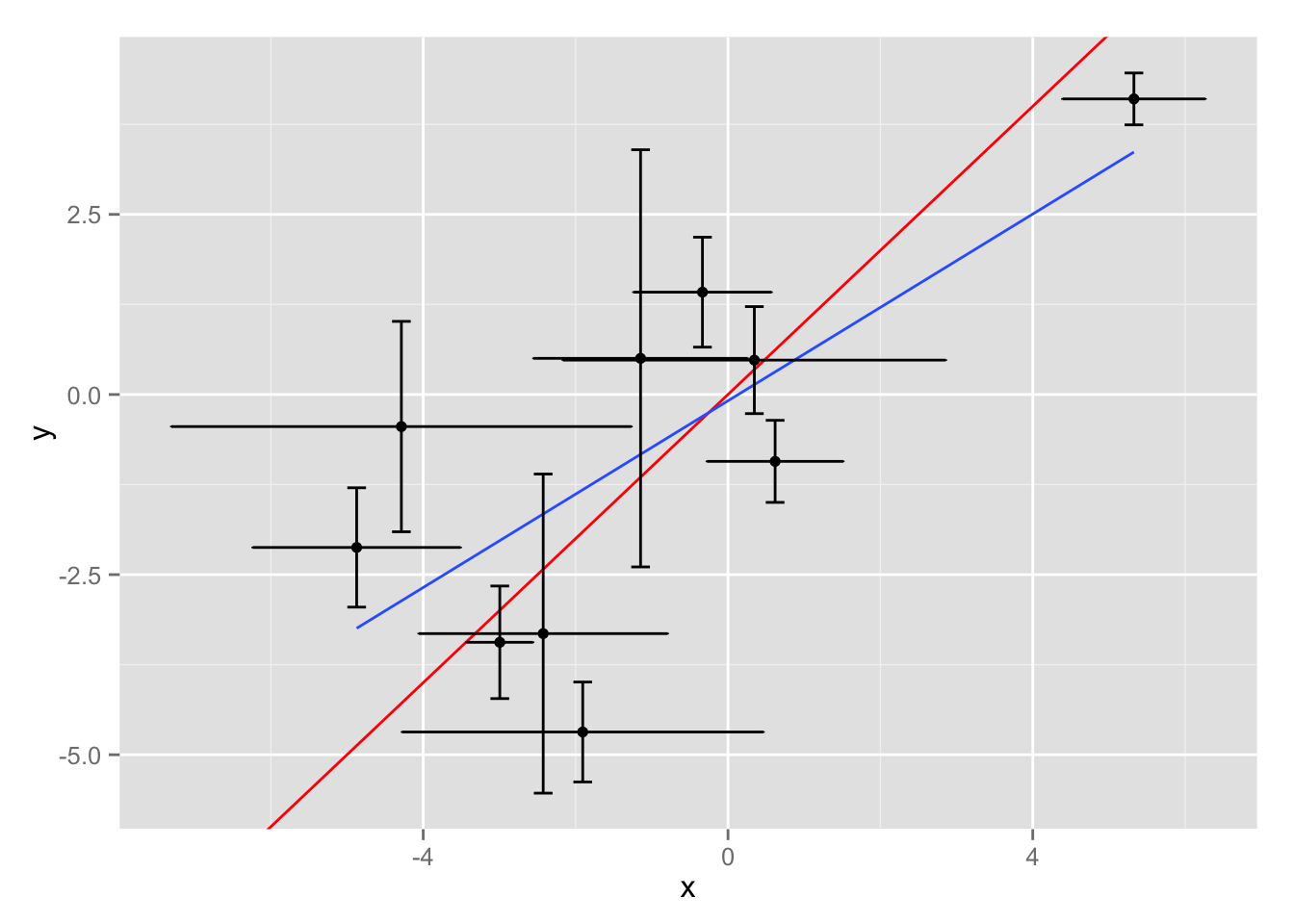(Red: actual dependency, blue: simple linear fit)

The simple linear model in STAN:

``````simple_code <- "
data {
int<lower=0> N;

vector[N] x;
vector[N] y;
}

parameters {
real slope;
real intercept;
real<lower=0> sigma;
}

model {
slope ~ normal(0, 10);
intercept ~ normal(0, 10);
sigma ~ cauchy(0, 25);

y ~ normal(x*slope+intercept, sigma);
}
"``````

And another model, taking uncertainty into account. Here, based on the proposed slope and intercept, the probability density function is maximized for each point:

``````uncertainty_code <- "
data {
int<lower=0> N;

vector[N] x;
vector[N] y;
vector<lower=0>[N] sigma_x;
vector<lower=0>[N] sigma_y;
}

parameters {
real slope;
real intercept;
}

transformed parameters {
vector[N] xx;
vector[N] yy;

vector[N] sx2;
vector[N] sy2;

sx2 <- sigma_x .* sigma_x;
sy2 <- sigma_y .* sigma_y;

// find maximum PDF given slope and intercept
xx <- ( (sy2 .* x) + slope * sx2 .* ( y - intercept ) ) ./ ( slope * sx2 + sy2);
yy <- slope * xx + intercept;
}

model {
slope ~ normal(0, 10);
intercept ~ normal(0, 10);

xx ~ normal(x, sigma_x);
yy ~ normal(y, sigma_y);
}
"``````

Let’s generate fits:

``````fit <- stan(model_code = uncertainty_code, data = data_list)
fit_simple <- stan(model_code = simple_code, data = data_list)``````

Comparing the parameter distributions, taking uncertainty into account produces a much better fit:

``````slopes <- do.call(cbind, extract(fit, "slope"))
intercepts <- do.call(cbind, extract(fit, "intercept"))

slopes_simple <- do.call(cbind, extract(fit_simple, "slope"))
intercepts_simple <- do.call(cbind, extract(fit_simple, "intercept"))

df_coef <- rbind(
data.frame( kind = "simple", slope = slopes_simple, intercept = intercepts_simple ),
data.frame( kind = "uncertainty", slope = slopes, intercept = intercepts )
)

ggplot(df_coef, aes(slope, color=kind)) + geom_density()``````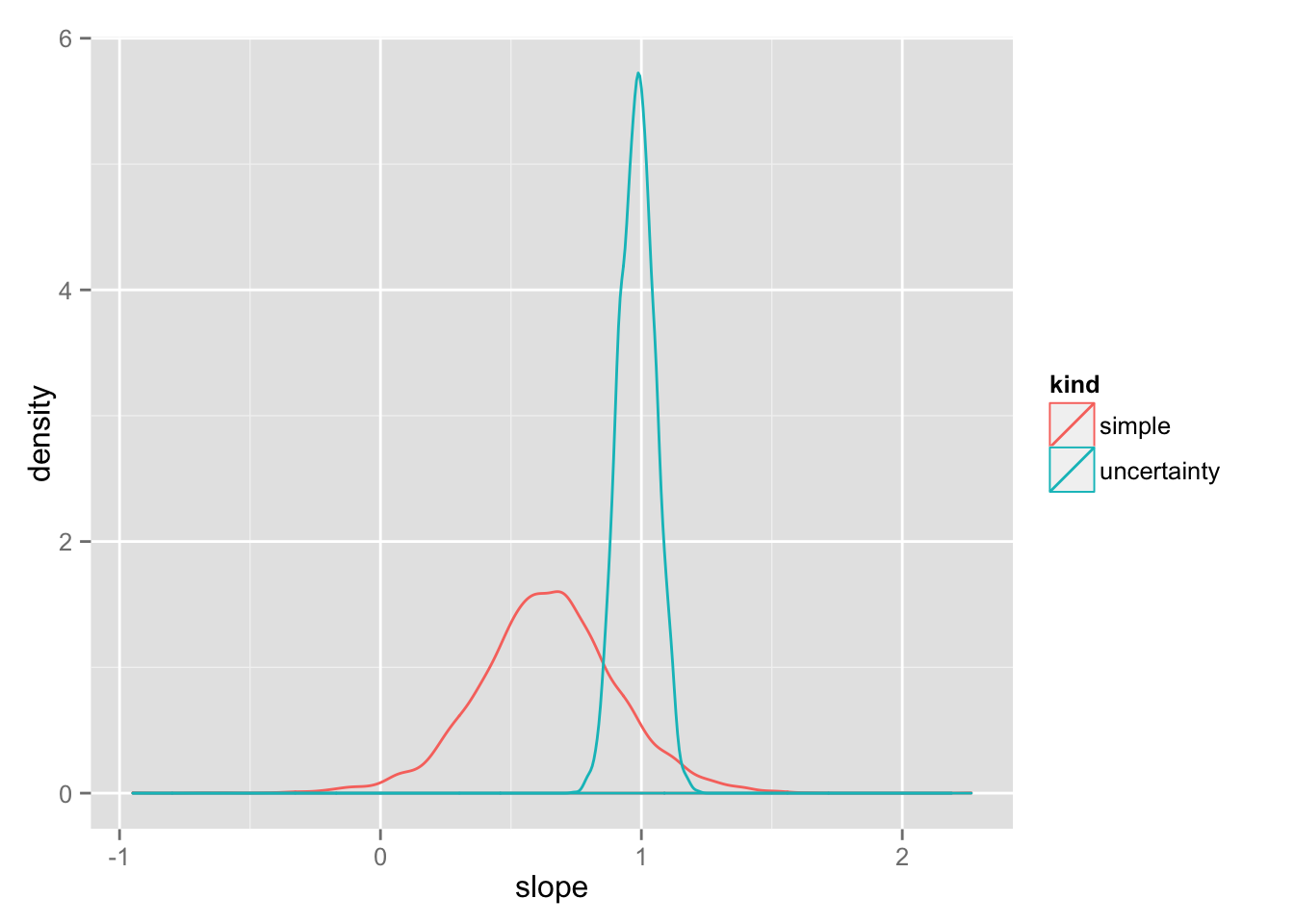``ggplot(df_coef, aes(intercept, color=kind)) + geom_density()``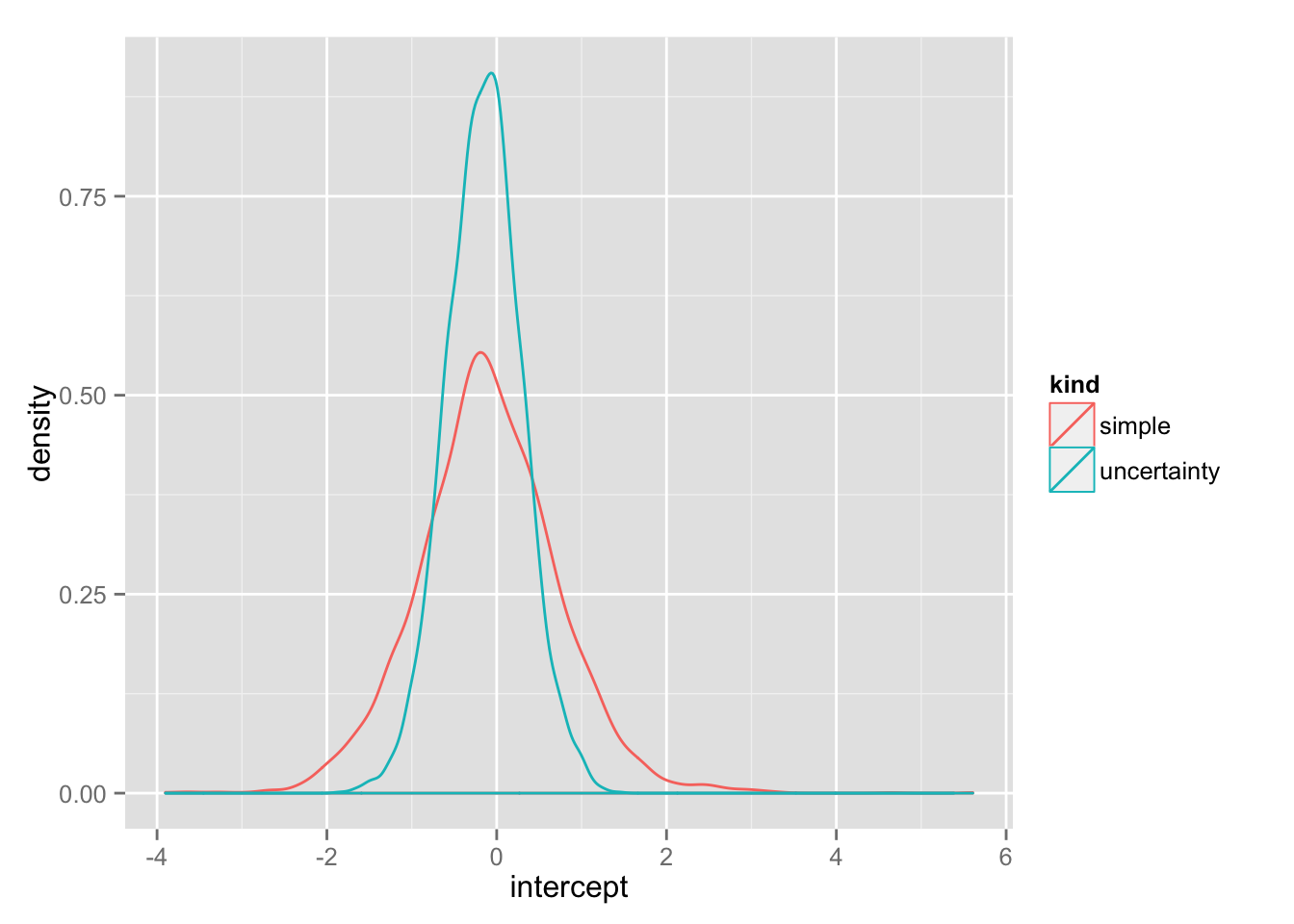Here are some sample fits:

``````samples <- sample.int(length(slopes), 50)
df_lines <- data.frame(slope=slopes[samples], intercept=intercepts[samples])

ggplot( data, aes(x, y) ) + geom_point() + geom_abline(data=df_lines, aes(slope=slope, intercept=intercept), color="grey") + coord_fixed() + geom_abline(color="red") + geom_smooth(method="lm", se=F)``````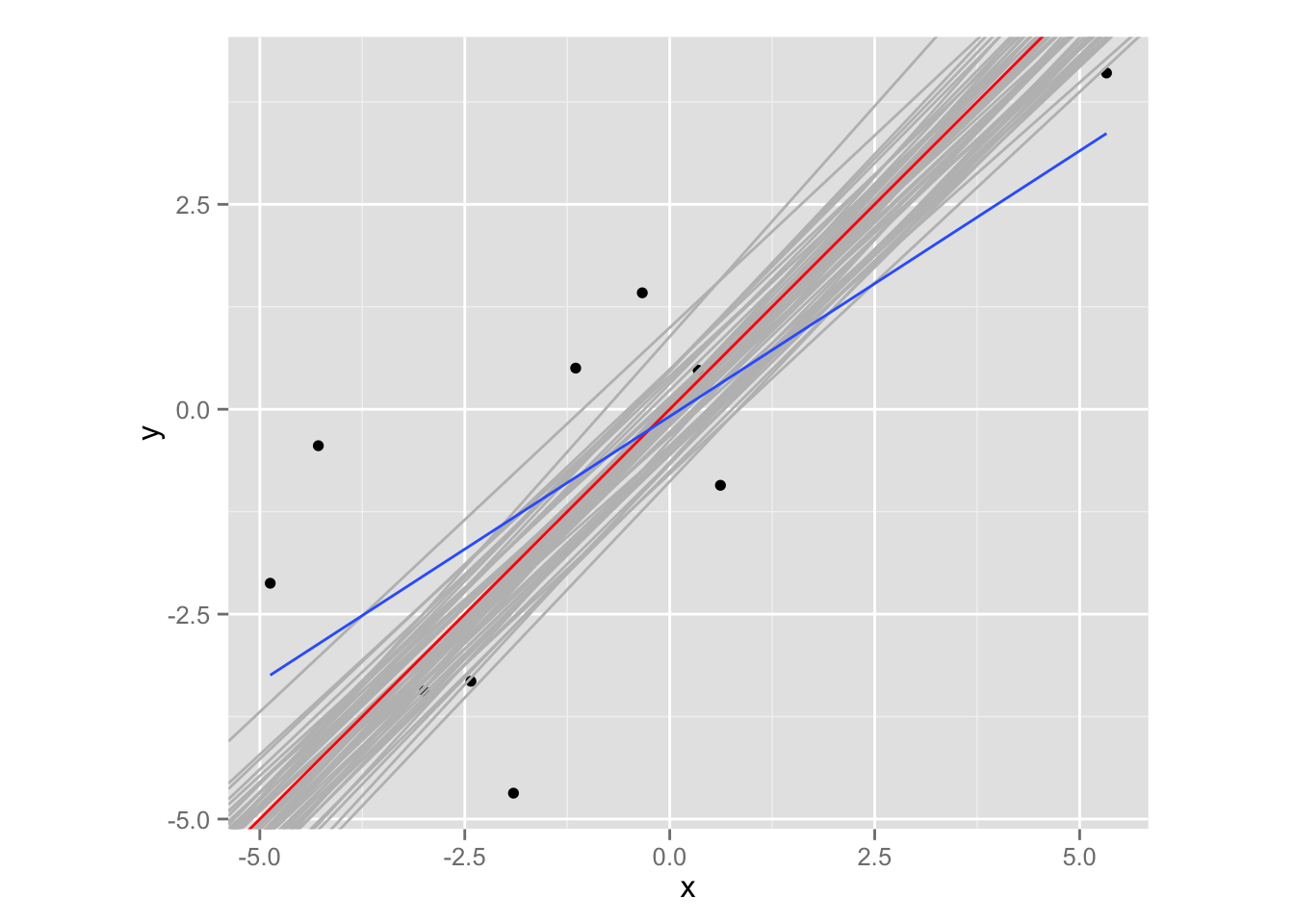And a view of the “moved” points:

``````xx <- do.call(cbind, extract(fit, "xx"))
yy <- do.call(cbind, extract(fit, "yy"))

df_points <- data.frame( x=c(x, xx[samples,]), y=c(y, yy[samples,]), kind = rep( c("measured", "inferred"), each=N), group = rep(1:N,2)  )

ggplot( df_points, aes(x, y, group=group)) + geom_point(aes(color=kind)) + geom_path() + geom_abline(color="green") + coord_fixed()``````